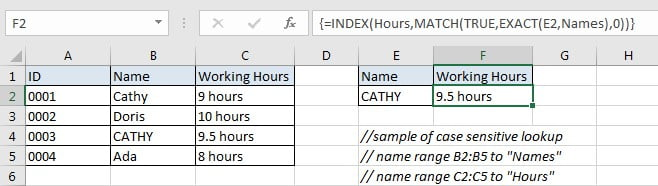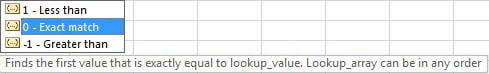# Basic Usage of INDEX & MATCH – Case Sensitive Lookup

In Excel, INDEX function and MATCH function are often used together for retrieving data from a particular position. MATCH function is one of Excel lookup & reference functions that can perform approximate match or exact match by setting different match types. They are not case-sensitive functions. If we need to perform a case-sensitive lookup, we need help from other functions.

Today, we will show you the usage of INDEX and MATCH with the help of EXACT function to perform a case-sensitive lookup. This formula will help you to retrieve data from a particular position of a given table based on the input lookup value exactly (case-sensitive). If you meet similar scenarios in your daily work, you can directly use this formula to deal with your problem.

## EXAMPLEIn this example, “Cathy” and “CATHY” are duplicate with different HR ID. To calculate working hours for “CATHY” correctly, we need to ignore “Cathy” and find out “CATHY” exactly, then retrieve correct working hours “9.5 hours” from cell C4 properly. To solve this problem, we can create a formula that can perform case-sensitive lookup and retrieves data from particular position based on the lookup value.

Before creating the formula, to make cell reference and range reference used in the formula are easy to understand, we named range (B2:B5) to “Names”, (C2:C5) to “Hours”. In this example, we will apply INDECT & MATCH functions together to perform an exact match. To perform case-sensitive lookup, we also apply EXACT function together with above two functions to help us.

## ANALYSIS

The formula must meet the following conditions:

1. It can scan values from “Names” and find out lookup value “CATHY” properly.

2. As we have two “Cathy” in “Names”, we need to ignore the first one as except the first letter, others are entered in lowercase. But for our input value “CATHY”, all five letters are entered in UPPERCASE. They are different if case sensitive.

3. After confirming the position of “CATHY” in range “Names”, it can retrieve data from the position just adjacent to “CATHY” in range “Hours”.

4. It returns proper working hours get in step#3 after performing the formula.

Actually, there are some powerful functions that can look up value effectively and powerfully, for example VLOOKUP function, we can use it to figure out most lookup cases in Excel. Besides, we can also apply function combinations to lookup values, for example INDEX and MATCH functions are good partners for retrieving data from a particular position. MATCH function can return the relative position of lookup value from a given table, and INDEXT can retrieve the data which is corresponding to the input value according to the position. And in Excel EXACT function can determine if strings are the same due to it is case sensitive. So, we will apply INDEX and MATCH together with EXACT function to create the formula to perform case-sensitive lookup:

`=INDEX(Hours,MATCH(TRUE,EXACT(E2,Names),0))`

## FORMULA

Input formula =INDEX(Hours,MATCH(TRUE,EXACT(E2,Names),0)) into F2, as this is an array formula, we must press Control+Shift+Enter, verify that “9.5 hours” is returned properly.In Excel 365, you can directly press Enter.

## FUNCTION INTRODUCTION

a.INDEX function returns a value or a reference based on given cell or range reference.

For array form:

Syntax:

=INDEX(array, row_num, [column_num])

For reference form:

Syntax:

`=INDEX(reference, row_num, [column_num], [area_num])`

There are two forms of INDEX functions. When applying INDEX with reference form, INDEX returns reference of the intersection of a particular row and column. You can apply array form if the first argument to INDEX is an array constant.

b. MATCH function returns the relative position of a cell from a range which cell contains specific item.

Syntax:

`=MATCH(lookup_value, lookup_array, [match_type])`

c. EXACT function is case-sensitive, it can detect whether two strings are exactly the same. If yes, it returns TRUE, else it returns FALSE.

Syntax:

`=EXACT(text1, text2)`

## EXPLANATION

`=INDEX(Hours,MATCH(TRUE,EXACT(E2,Names),0))`

a. EXACT(E2,Names) performs a case-sensitive comparison between value in E2 (“CATHY”) and values in “Names”({“Cathy”;”Doris”;”CATHY”;”Ada”}). It returns “TRUE” if the two strings are exactly the same. So, after comparison, we get below an array of “TRUE” and “FALSE”:

`{FALSE;FALSE;TRUE;FALSE}`

{FALSE;FALSE;TRUE;FALSE} is delivered to MATCH function as lookup_array directly. In this array, “TRUE” represents “CATHY”, its position in the array reflects the position of “CATHY” in range “Names”.

b. MATCH(TRUE,{FALSE;FALSE;TRUE;FALSE},0) returns the relative position of input lookup value “TRUE” from array {FALSE;FALSE;TRUE;FALSE} which is returned by EXACT. As “TRUE” is at the third position in the array, so MATCH returns 3.

There are three match types, to perform an exact match comparison, we set match type to 0.c. Above result “3” is delivered to INDEX function as row number. Expand values in “Score”. INDEX(Hours,3) returns the value in the third row of range “Hours” as the final result.

`=INDEX({"9 hours";"10 hours";"9.5 hours";"8 hours"},3)`

// returns “9.5 hours” finally

### Related Functions

• Excel INDEX function
The Excel INDEX function returns a value from a table based on the index (row number and column number)The INDEX function is a build-in function in Microsoft Excel and it is categorized as a Lookup and Reference Function.The syntax of the INDEX function is as below:= INDEX (array, row_num,[column_num])…
• Excel MATCH  function
The Excel MATCH function search a value in an array and returns the position of that item.The MATCH function is a build-in function in Microsoft Excel and it is categorized as a Lookup and Reference Function.The syntax of the MATCH function is as below:= MATCH  (lookup_value, lookup_array, [match_type])….
• Excel EXACT function
The Excel EXACT function compares if two text strings are the same and returns TRUE if they are the same, Or, it will return FALSE.The syntax of the EXACT function is as below:= EXACT (text1,text2)…